Note: The other languages of the website are Google-translated. Back to English

## How to sumif with one or more criteria in Excel?

In Excel, sum values based on one or more criteria is a common task for most of us, the SUMIF function can help us to quickly sum the values based on one condition and the SUMIFS function help us to sum values with multiple criteria. This article, I will describe how to sum with one or more criteria in Excel?

Sum cell values with one criterion in Excel

Sum cell values with multiple criteria in Excel

####Sum cell values with one criterion in Excel

First, I will give the SUMIF syntax to you for reference:

SUMIF(range, criteria, [sum_range])

• Range: the range of cells to be evaluated by your criteria;
• Criteria: the condition that you want to use;
• Sum_range: the range of cells to sum if the condition is met

For applying this SUMIF function, I will take the following data for example: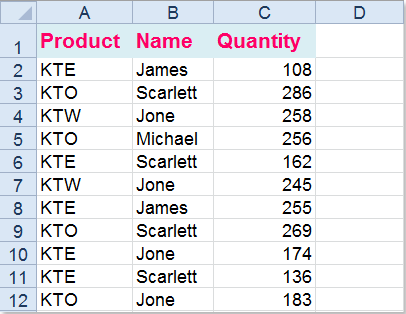(1.) Sum of quantity when the product is “KTE”:

=SUMIF(A2:A12,E2,C2:C12) and press Enter key to get the result, (A2:A12 is the range cells which contains the criteria, E2 is the criterion you want to sum the values based on, C2:C12 refers to the range you want to sum) see screenshot: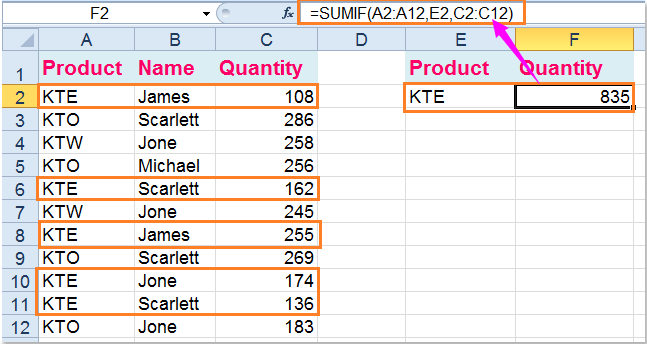=SUMIF(A2:A12,"<>KTE",C2:C12), then press Enter key to add up all product quantity excluding KTE, (A2:A12 is the range cells which contains the criteria, <>KTE is the criterion which excludes KTE, C2:C12 refers to the range you want to sum), see screenshot: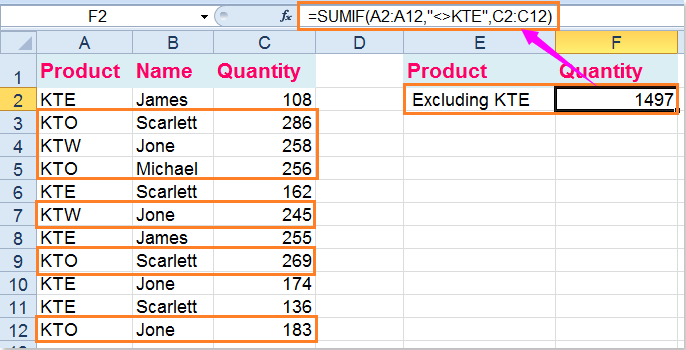(3.) Sum the quantity greater than 250:

=SUMIF(C2:C12,">250"), and press Enter key to get the result, (C2:C12 refers to the range you want to sum, >250 is the criterion you need, you also can change it to less than 250 as you need), see screenshot: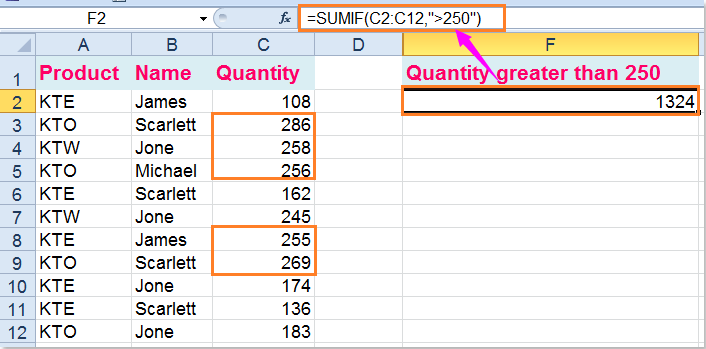(4.) Use SUMIF+SUMIF+… function with multiple OR criteria, sum the quantity of KTE and KTW:

=SUMIF(A2:A12,"KTE",C2:C12) + SUMIF(A2:A12,"KTW",C2:C12)( A2:A12 is the range cells which contains the criteria, KTE and KTW are the criteria you want to sum the values based on, C2:C12 refers to the range you want to sum), and then press Enter key to get the result: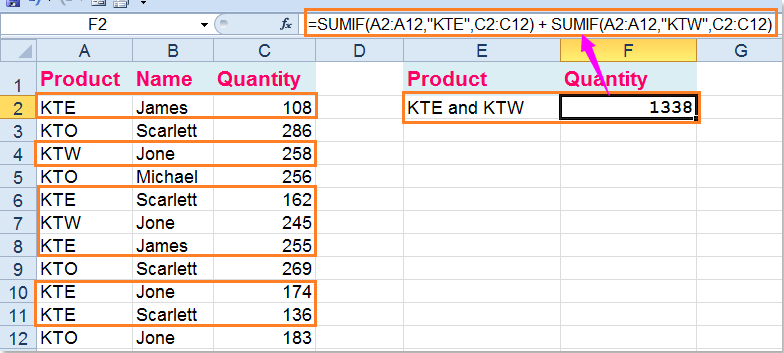####Sum cell values with multiple criteria in Excel

The SUMIFS syntax as follows:

SUMIFS(sum_range, criteria_range1, criteria1,[criteria_range2, criteria2],...)

• Sum_range: a range of cells to sum;
• Criteria_range1: the first range which has the criteria;
• criteria1: the first condition that must be met;
• criteria_range2, criteria2, ...: these are additional ranges and criteria associated with them.

(1.) Sum the quantity where the product is KTE and name is James:

Please enter this formula: =SUMIFS(C2:C12,A2:A12,"KTE",B2:B12,"James"),(C2:C12 is the range of cells need to sum, A2:A12 , KTE are the first criteria range and criterion, B2:B12, James, are the second criteria range and criterion), and then press Enter key, see screenshot: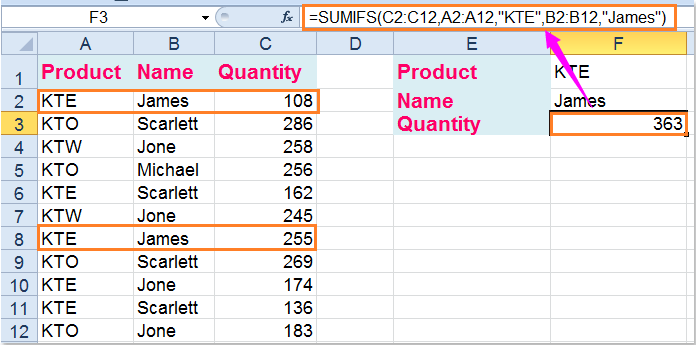Here also has an array formula can help you: =SUM((A2:A12="kte")*(B2:B12="James")*C2:C12), and you need to press Ctrl + Shift + Enter keys together after typing the formula.

(2.) Sum the quantity where the product is KTE and name is not James

Type this formula: =SUMIFS(C2:C12,A2:A12,"KTE",B2:B12,"<>James")(C2:C12 is the range of cells need to sum, A2:A12 , KTE are the first criteria range and criterion, B2:B12, <>James, are the second criteria range and criterion), then press Enter key to get the result, see screenshot: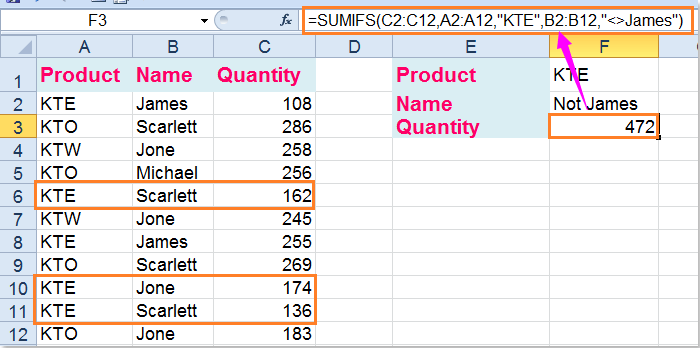Another array formula also can finish this operation: =SUM((A2:A12="kte")*(B2:B12<>"James")*C2:C12), but you must press Ctrl + Shift + Enter keys after typing this formula.

(3.) Sum the quantity where the product is KTE and quantity greater than 150

You can use this SUMIFS formula: =SUMIFS(C2:C12,A2:A12,"KTE",C2:C12,">150")(C2:C12 is the range of cells need to sum, A2:A12 , KTE are the first criteria range and criterionj, C2:C12, >150, are the second criteria range and criterion), and then tap the Enter key to return the result.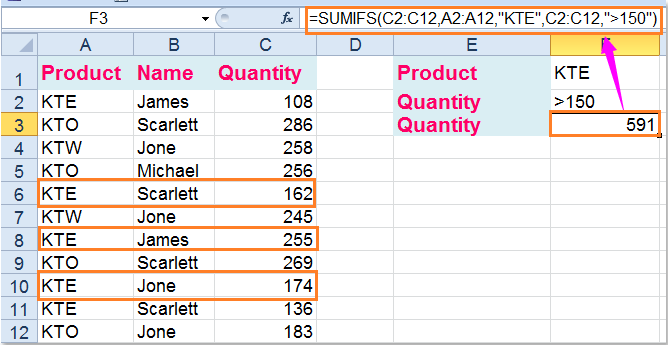You can also us the SUM array formula to get the result you want, =SUM((A2:A12="kte")*(C2:C12>150)*C2:C12) please remember to press Ctrl + Shift + Enter keys after typing this formula.

(4.) Sum the quantity which quantity between 100 and 200

To sum the values between two numbers, please use this formula: =SUMIFS(C2:C12,C2:C12,">100",C2:C12,"<200")( C2:C12 is the range of cells need to sum, C2:C12 , >100 are the first criteria range and criteria, C2:C12, <200, are the second criteria range and criteria), and then press Enter key to get the result as follows: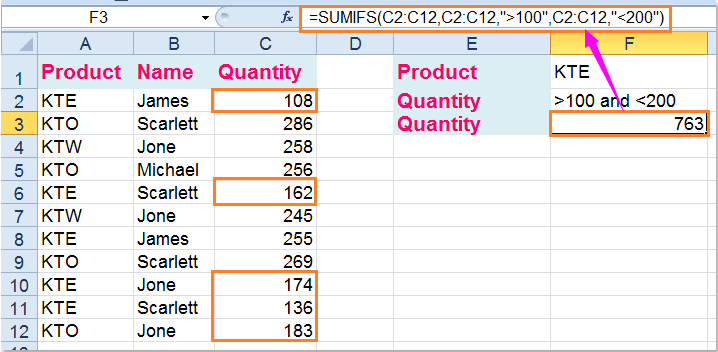Of course, a SUM array formula can help you as well, =SUM((C2:C12>100)*(C2:C12<200)*C2:C12), remember to press Ctrl + Shift + Enter keys together to get the right result.

Related articles:

How to sum multiple columns based on single criteria in Excel?

How to sum cells with text and numbers in Excel?

### The Best Office Productivity Tools

#### Kutools for Excel Solves Most of Your Problems, and Increases Your Productivity by 80%

• Reuse: Quickly insert complex formulas, charts and anything that you have used before; Encrypt Cells with password; Create Mailing List and send emails...
• Super Formula Bar (easily edit multiple lines of text and formula); Reading Layout (easily read and edit large numbers of cells); Paste to Filtered Range...
• Merge Cells/Rows/Columns without losing Data; Split Cells Content; Combine Duplicate Rows/Columns... Prevent Duplicate Cells; Compare Ranges...
• Select Duplicate or Unique Rows; Select Blank Rows (all cells are empty); Super Find and Fuzzy Find in Many Workbooks; Random Select...
• Exact Copy Multiple Cells without changing formula reference; Auto Create References to Multiple Sheets; Insert Bullets, Check Boxes and more...
• Extract Text, Add Text, Remove by Position, Remove Space; Create and Print Paging Subtotals; Convert Between Cells Content and Comments...
• Super Filter (save and apply filter schemes to other sheets); Advanced Sort by month/week/day, frequency and more; Special Filter by bold, italic...
• Combine Workbooks and WorkSheets; Merge Tables based on key columns; Split Data into Multiple Sheets; Batch Convert xls, xlsx and PDF...
• More than 300 powerful features. Supports Office / Excel 2007-2021 and 365. Supports all languages. Easy deploying in your enterprise or organization. Full features 30-day free trial. 60-day money back guarantee.#### Office Tab Brings Tabbed interface to Office, and Make Your Work Much Easier

• Enable tabbed editing and reading in Word, Excel, PowerPoint, Publisher, Access, Visio and Project.
• Open and create multiple documents in new tabs of the same window, rather than in new windows.
• Increases your productivity by 50%, and reduces hundreds of mouse clicks for you every day!